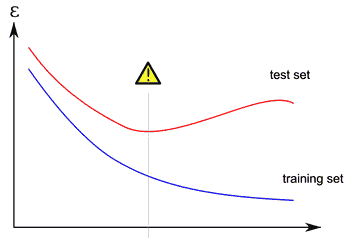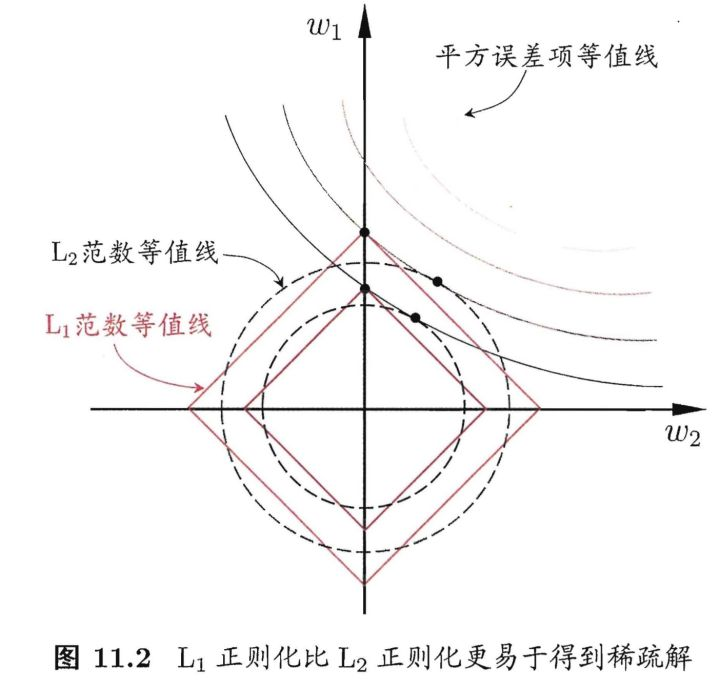# 正则化方法：数据增强、regularization、dropout

## 过拟合 overfitting

• 在训练数据不够多，或者模型过于复杂时，常常会导致模型对训练数据集过度拟合。

• 其直观的表现如下图所示：随着训练过程的进行，在训练集上的错误率渐渐减小，但是在验证集上的错误率却反而渐渐增大。• 正则化技术是保证算法泛化能力的有效工具。

## 正则化项

$J(w,b)= \frac{1}{m} \sum_{i=1}^{m}L(\hat{y},y)+\lambda R(w)$

• $L(\hat{y},y)$ 是经验风险项

• $\lambda R(w)$ 是正则项

• $λ≥0$ 为调整两者之间关系的系数

• $λ$ 值可以使用交叉验证的方法尝试一系列可能的值，比如从 0，0.01，0.02，0.04 … 开始，一直试下去，将步长设为2倍的速度增长。

## 范数 norm

• $L_1$ 范数： $||x||_1 = \sum_{i=1}^N|x_i|$

即向量元素绝对值之和。

• $L_2$ 范数： $||\textbf{x}||_2 =\sqrt{\sum_{i=1}^Nx_i^2}$

即 Euclid范数（欧几里得范数），常用计算向量长度。

• $L_p$ 范数：$||\textbf{x}||_p = (\sum_{i=1}^N|x_i|^p)^{\frac{1}{p}}$

即向量元素绝对值的 $p$ 次方之和的 $1/p$ 次幂

## L1 正则 Lasso regularizer

$J(w,b)=\frac{1}{m} \sum_{i=1}^{m}L(\hat{y},y)+\lambda|w|$

• L1正则化，是一个相对常用的正则化方法。

• 正则化目的：减少参数的绝对值总和。

## L2 正则 Ridge Regularizer / Weight Decay

$J(w,b)=\frac{1}{m} \sum_{i=1}^{m}L(\hat{y},y)+\frac{1}{2}\lambda w^2$

• L2 正则化，可能是最常用的正则化方法了

• 正则化目的：减少参数的平方值总和。

• 系数 $\frac{1}{2}$ 主要是为了后面的求导操作方便，加上 $\frac{1}{2}$ 后，该式子关于 $w$ 梯度就是 $\lambda w$ 而不是 $2\lambda w$ 了。

• L2 正则化，可以直观理解为它对于大数值的权重向量进行严厉惩罚，倾向于更加分散的权重向量，使网络更倾向于使用所有输入特征，而不是严重依赖输入特征中某些小部分特征。

举个例子，假设输入向量 $x=[1,1,1,1]$，两个权重向量 $w_1=[1,0,0,0]，w_2=[0.25,0.25,0.25,0.25]$。那么 $w^T_1x=w^T_2=1$，两个权重向量都得到同样的内积，但是 $w_1$ 的 L2 惩罚是 $1.0$，而 $w_2$ 的 L2 惩罚是 $0.25$

因此，根据 L2 惩罚来看，$w_2$ 更好，因为它的正则化损失更小。从直观上来看，这是因为 $w_2$ 的权重值更小且更分散。既然 $L2$ 惩罚倾向于更小更分散的权重向量，这就会鼓励分类器最终将所有维度上的特征都用起来，而不是强烈依赖其中少数几个维度。

• 在梯度下降时，使用 L2 正则化意味着所有的权重都以 $w$ += - $\lambda *w$ 向着 0 线性下降。

## L1 与 L2 的差异L1 与 L2 的差异

• 假设个体 $x$ 只有两个分量，则 $w$ 也只有两个分量 $w1，w2$，将其作为两个坐标轴，对于目标函数：

$J(w,b)= \frac{1}{m} \sum_{i=1}^{m}(y_i -w^Tx_i)^2+\lambda R(w)$

• 先绘出目标函数中 $(y_i -w^Tx_i)^2$ 的平方误差项等值线（平方误差项取值相同的点的连线）。

• 再分别绘制出 L1 范数和 L2 范数的等值线。

• 目标函数的，要在平方误差项与正则化项之间折中，即出现在图中的等值线交点处。

• 可发现采用 L1 范数时，平方误差等值线与正则化等值线的交点经常出现在坐标轴上，即 $w1$$w2$$0$

• 而采用 L2 范数时，交点经常出现在象限中，即 $w1$$w2$ 均不为 $0$，故采用 L1 正则化项更易得到稀疏解。

L1 与 L2 的使用

• 由于 L1 正则化得到的是稀疏解，它会让权重向量在最优化的过程中变得稀疏（即非常接近0），使用 L1 正则化的神经元最后使用的是它们最重要的输入特征的稀疏子集。

• 相较 L1 正则化，L2 正则化中的权重向量大多是分散的小数字。

• 在实践中，如果不是特别关注某些明确的特征选择，一般说来 L2 正则化都会比 L1 正则化效果好。

## Dropout

L1、L2 正则化是通过修改代价函数来实现的，而 Dropout 则是通过修改神经网络本身来实现的，它是在训练网络时用的一种技巧。• 在训练网络时，对于完整的网络结构（如左图），每次迭代时，都让神经元以超参数 $p$ 的概率被随机地停用（Dropout），即输出置为0，如右图。

• 在训练时，保持输入输出层不变，数据前向传播后，对得到的损失结果进行反向传播，更新神经网络中的权值时，不更新被停用的单元。

• 在预测时，不进行随机失活，但是神经元的输出都要乘以超参数 $p$，调整其数值范围。以 $p=0.5$ 为例，在预测时神经元必须把它们的输出减半，这是因为在训练的时候它们的输出只有一半。

""" 普通版随机失活: 不推荐实现 """

p = 0.5 # 激活神经元的概率. p值更高 = 随机失活更弱

def train_step(X):
""" X中是输入数据 """

# 3层neural network的前向传播
H1 = np.maximum(0, np.dot(W1, X) + b1)
U1 = np.random.rand(*H1.shape) < p # 第一个随机失活遮罩
H1 *= U1 # drop!
H2 = np.maximum(0, np.dot(W2, H1) + b2)
U2 = np.random.rand(*H2.shape) < p # 第二个随机失活遮罩
H2 *= U2 # drop!
out = np.dot(W3, H2) + b3

# 反向传播:计算梯度... (略)
# 进行参数更新... (略)

def predict(X):
# 前向传播时模型集成
H1 = np.maximum(0, np.dot(W1, X) + b1) * p # 注意：激活数据要乘以p
H2 = np.maximum(0, np.dot(W2, H1) + b2) * p # 注意：激活数据要乘以p
out = np.dot(W3, H2) + b3


• 相对于上述的随机失活，实际应用中更倾向使用反向随机失活（inverted dropout），它是在训练时就进行数值范围调整，从而让前向传播在测试时保持不变。

• 这样做还有一个好处，无论你决定是否使用随机失活，预测方法的代码可以保持不变。反向随机失活的代码如下：

"""

"""

p = 0.5 # 激活神经元的概率. p值更高 = 随机失活更弱

def train_step(X):
# 3层neural network的前向传播
H1 = np.maximum(0, np.dot(W1, X) + b1)
U1 = (np.random.rand(*H1.shape) < p) / p # 第一个随机失活遮罩. 注意/p!
H1 *= U1 # drop!
H2 = np.maximum(0, np.dot(W2, H1) + b2)
U2 = (np.random.rand(*H2.shape) < p) / p # 第二个随机失活遮罩. 注意/p!
H2 *= U2 # drop!
out = np.dot(W3, H2) + b3

# 反向传播:计算梯度... (略)
# 进行参数更新... (略)

def predict(X):
# 前向传播时模型集成
H1 = np.maximum(0, np.dot(W1, X) + b1) # 不用数值范围调整了
H2 = np.maximum(0, np.dot(W2, H1) + b2)
out = np.dot(W3, H2) + b3


Dropout 对过拟合的解决

• 取平均的作用：

用相同的训练数据去训练 n 个不同的神经网络，一般会得到 n 个不同的结果，此时我们可以采用 n 个结果取均值去决定最终结果。

取均值策略通常可以有效防止过拟合问题。因为不同的网络可能产生不同的过拟合，取均值则有可能让一些 “相反的” 拟合互相抵消。

dropout 随机停用不同的隐层神经元，导致在每次迭代时都使用了不同的网络结构，就类似在训练不同的网络，整个 dropout 过程就相当于对很多个不同的神经网络取平均。

• 减少神经元之间复杂的共适应关系：

因为 dropout 程序导致两个神经元不一定每次都在一个 dropout 网络中出现。这样权值的更新不再依赖于有固定关系的隐含节点的共同作用，阻止了某些特征仅仅在其它特定特征下才有效果的情况，迫使网络去学习更加鲁棒的特征。